• 一种新的最佳聚类数确定方法一种新的最佳聚类数确定方法摘要：为了更有效地确定数据集的聚类数最佳聚类数，提出一种新的确定数据集最佳聚类数的算法。该算法借签层次聚类的思想，一次性地生成所有可能的划分，然后...
一种新的最佳聚类数确定方法一种新的最佳聚类数确定方法摘要：为了更有效地确定数据集的聚类数最佳聚类数，提出一种新的确定数据集最佳聚类数的算法。该算法借签层次聚类的思想，一次性地生成所有可能的划分，然后根据有效性指标选择最佳的聚类划分，进而获得最佳聚类数。理论分析和实验结果证明，该算法具有良好的性能。关键词：层次聚类；最佳聚类数；聚类有效性指标；聚类最佳聚类数的判定通常采用一种基于迭代的trial-and-error过程进行，该过程是在给定的数据集上，使用不同的参数(通常是聚类数k),运行特定的聚类算法,对数据集进行不同的划分，然后计算每种划分的指标值。通过比较各个指标值，其中符合预定条件的指标值所对应的聚类个数被认为是最佳的聚类数。实际上，trial-and-error过程存在两个不足之处:(1)聚类数k值的确定对于缺乏丰富聚类分析经验的用户来说是难以准确确定的，这需进一步提出寻找更合理的聚类数k的方法;(2)目前提出的许多检验聚类有效性的指标，如Vxie指标、Vwsj指标等，但这些指标都是基于某个特定聚类算法提出的，在实际应用中受到了极大限制。鉴于上述两种情况，本文借鉴层次聚类的思想一次性地生成所有可能的聚类划分，并计算其对应的有效性指标，然后选择指标值最小的聚类划分来估计数据集的最佳聚类数，这样可以避免对大型数据集的反复聚类，而且该过程不依赖于特定的聚类算法。1聚类有效性指标本文采用的是一个不依赖于具体算法的有效性指标Q(C)来评估数据集的聚类效果。该有效性指标主要是通过类内数据对象的紧凑度以及类间数据对象的分离度衡量聚类质量。1.3噪声点与孤立点的消除基于数据集中存在的噪声点与孤立点对聚类结果的影响，本文认为单独利用有效性指标所得出的聚类数为最佳聚类数k*的结论并不成立。根据
展开全文• 为了更有效地确定数据集的最佳聚类数，提出一种新的确定数据集最佳聚类数的算法。该算法借签层次聚类的思想，一次性地生成所有可能的划分，然后根据有效性指标选择最佳的聚类划分，进而获得最佳聚类数。理论分析和...软件
• 在聚类分析中, 决定聚类质量的关键是确定最佳聚类数. 对此, 从样本几何结构的角度定义了样本聚类距离 和样本聚类离差距离, 设计了一种新的聚类有效性指标. 在此基础上, 提出一种基于近邻传播算法确定样本最佳...
• 在确定最佳聚类数的过程中采用K-means算法，针对K-means算法随机选择初始聚类中心的缺陷，提出以欧式距离度量样本相似度，基于样本方差，选出方差最小的前K个样本作为初始聚类中心，避免噪声点成为初始聚类中心，...
• 另一种是内部指标，用于评价同一聚类算法在不同聚类数条件下聚类结果的优良程度，通常用来确定数据集的最佳聚类数。 一 最佳聚类数判定的方法 对于内部指标，通常分为三种类型：基于数据集模糊划分的指标；基于...
聚类有效性的评价标准有两种：一是外部标准，通过测量聚类结果和参考标准的一致性来评价聚类结果的优良；另一种是内部指标，用于评价同一聚类算法在不同聚类数条件下聚类结果的优良程度，通常用来确定数据集的最佳聚类数。 一 最佳聚类数判定的方法
对于内部指标，通常分为三种类型：基于数据集模糊划分的指标；基于数据集样本几何结构的指标；基于数据集统计信息的指标。基于数据集样本几何结构的指标根据数据集本身和聚类结果的统计特征对聚类结果进行评估，并根据聚类结果的优劣选取最佳聚类数，这些指标有Calinski-Harabasz(CH)指标，Davies-Bouldin(DB)指标Weighted inter-intra(Wint)指标，Krzanowski-Lai(KL)指标，Hartigan(Hart)指标，In-Group Proportion(IGP)指标等。本文主要介绍Calinski-Harabasz(CH)指标和Davies-Bouldin(DB)指标。
（1） CH指标
CH指标通过类内离差矩阵描述紧密度，类间离差矩阵描述分离度，指标定义为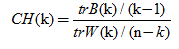其中，n表示聚类的数目 ,k 表示当前的类, trB(k)表示类间离差矩阵的迹, trW(k) 表示类内离差矩阵的迹。有关公式更详细的解释可参考论文“ A  dendrite  method  for  cluster  analysis ”。
可以得出 CH越大代表着类自身越紧密，类与类之间越分散，即更优的聚类结果。

（2） DB指标
DB指标通过描述样本的类内散度与各聚类中心的间距，定义为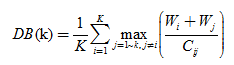其中，K是聚类数目，Wi表示类Ci中的所有样本到其聚类中心的平均距离，Wj表示类Ci中的所有样本到类Cj中心的平均距离，Cij表示类Ci和Cj中心之间的距离。可以看出，DB越小表示类与类之间的相似度越低，从而对应越佳的聚类结果。

最佳聚类数的确定过程一般是这样的：给定K 的范围[Kmin,Kmax]，对数据集使用不同的聚类数K运行同一聚类算法，得到一系列聚类结果，对每个结果计算其有效性指标的值，最后比较各个指标值，对应最佳指标值的聚类数即为最佳聚类数。

二 实验结果
在Matlab中，函数evalclusters提供了四种方法进行聚类效果的评估，包括’CalinskiHarabasz’ 、 ‘DaviesBouldin’ 、’gap’ 、 ‘silhouette’。选取一组数据，进行聚类效果评估。这里分别选用’CalinskiHarabasz’ 、 ‘DaviesBouldin’指标，聚类算法选择k-means.

（1） CH指标
给定K值范围1~3，计算每个聚类结果的CH 指标，最大指标值对应的K 值为最优值。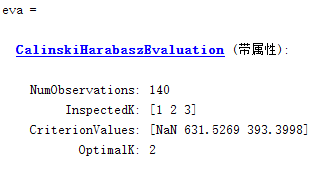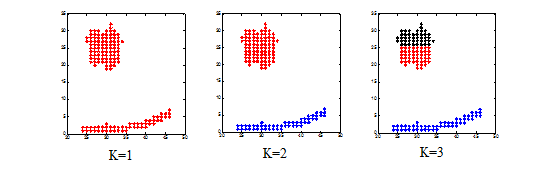（2） DB指标
给定K值范围1~3，计算每个聚类结果的DB 指标，最大指标值对应的K 值为最优值。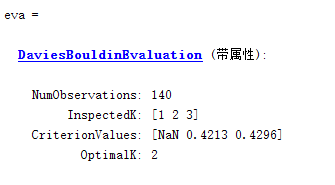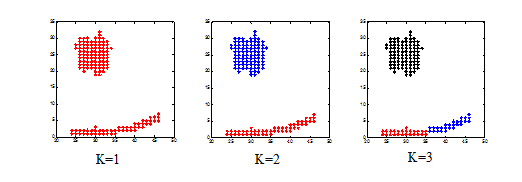备注：出现NAN是因为这两种方法均不适用与聚类数为1的情况。

Matlab代码

cluster = zeros(size(data,1),3);
for i=1:3
cluster(:,i) = kmeans(data,i,'replicate',5); %%%保存每次聚类结果
end
eva = evalclusters(data,cluster,'DaviesBouldin');

subplot(1,3,1);
plot(data(cluster(:,1)==1,1),data(cluster(:,1)==1,2),'r*');
hold on

subplot(1,3,2);
plot(data(cluster(:,2)==1,1),data(cluster(:,2)==1,2),'r*');
hold on
plot(data(cluster(:,2)==2,1),data(cluster(:,2)==2,2),'b*');
hold on

subplot(1,3,3);
data=[c1 r1];
[idx,ctrs] = kmeans(data,3);
plot(data(cluster(:,3)==1,1),data(cluster(:,3)==1,2),'r*');
hold on
plot(data(cluster(:,3)==2,1),data(cluster(:,3)==2,2),'b*');
hold on
plot(data(cluster(:,3)==3,1),data(cluster(:,3)==3,2),'k*');
hold on


展开全文• K均值聚类 在这个项目中，我使用K均值聚类为虹膜数据搜索了最佳聚类数
• 提出了一种复杂系统最佳聚类数的确定方法，首先检验系统的可聚性，然后给定合理的聚类数区间，最后在聚类数区间中搜寻得到最佳聚类数，其中搜寻依据为尽可能满足经典的聚类有效性指标。对X30及Bensaid数据检验及全国...聚类有效性函数
• 利用FCM求解最佳聚类数的算法中，每次应用FCM算法都要重新初始化类中心，而FCM算法对初始类中心敏感，这样使得利用FCM求解最佳聚类数的算法很不稳定。对该算法进行了改进，提出了一个合并函数，使得（c－1）类的类...
• 原文链接：http://tecdat.cn/?p=7275​tecdat.cn确定数据集中最佳的簇数是分区聚类（例如k均值聚类）中的一个基本... 我们将介绍用于确定k均值，k medoids（PAM）和层次聚类的最佳聚类数的不同方法。这些方法包括直...
原文链接：http://tecdat.cn/?p=7275​tecdat.cn确定数据集中最佳的簇数是分区聚类（例如k均值聚类）中的一个基本问题，它要求用户指定要生成的簇数k。一个简单且流行的解决方案包括检查使用分层聚类生成的树状图，以查看其是否暗示特定数量的聚类。不幸的是，这种方法也是主观的。 我们将介绍用于确定k均值，k medoids（PAM）和层次聚类的最佳聚类数的不同方法。这些方法包括直接方法和统计测试方法：直接方法：包括优化准则，例如簇内平方和或平均轮廓之和。相应的方法分别称为弯头方法和轮廓方法。统计检验方法：包括将证据与无效假设进行比较。 除了肘部，轮廓和间隙统计方法外，还有三十多种其他指标和方法已经发布，用于识别最佳簇数。我们将提供用于计算所有这30个索引的R代码，以便使用“多数规则”确定最佳聚类数。对于以下每种方法：我们将描述基本思想和算法我们将提供易于使用的R代码，并提供许多示例，用于确定最佳簇数并可视化输出。肘法回想一下，诸如k-均值聚类之类的分区方法背后的基本思想是定义聚类，以使总集群内变化[或总集群内平方和（WSS）]最小化。总的WSS衡量了群集的紧凑性，我们希望它尽可能小。Elbow方法将总WSS视为群集数量的函数：应该选择多个群集，以便添加另一个群集不会改善总WSS。最佳群集数可以定义如下：针对k的不同值计算聚类算法（例如，k均值聚类）。例如，通过将k从1个群集更改为10个群集。对于每个k，计算群集内的总平方和（wss）。根据聚类数k绘制wss曲线。曲线中拐点（膝盖）的位置通常被视为适当簇数的指标。平均轮廓法平均轮廓法计算不同k值的观测值的平均轮廓。聚类的最佳数目k是在k的可能值范围内最大化平均轮廓的数目（Kaufman和Rousseeuw 1990）。差距统计法 该方法可以应用于任何聚类方法。间隙统计量将k的不同值在集群内部变化中的总和与数据空引用分布下的期望值进行比较。最佳聚类的估计将是使差距统计最大化的值（即，产生最大差距统计的值）。 资料准备我们将使用USArrests数据作为演示数据集。我们首先将数据标准化以使变量具有可比性。head(df) ##            Murder Assault UrbanPop     Rape ## Alabama    1.2426   0.783   -0.521 -0.00342 ## Alaska     0.5079   1.107   -1.212  2.48420 ## Arizona    0.0716   1.479    0.999  1.04288 ## Arkansas   0.2323   0.231   -1.074 -0.18492 ## California 0.2783   1.263    1.759  2.06782 ## Colorado   0.0257   0.399    0.861  1.86497  Silhouhette和Gap统计方法简化格式如下： 下面的R代码确定k均值聚类的最佳聚类数：# Elbow method fviz_nbclust(df, kmeans, method = "wss") +     geom_vline(xintercept = 4, linetype = 2)+   labs(subtitle = "Elbow method")  # Silhouette method  # Gap statistic## Clustering k = 1,2,..., K.max (= 10): .. done
## Bootstrapping, b = 1,2,..., B (= 50)  [one "." per sample]:
## .................................................. 50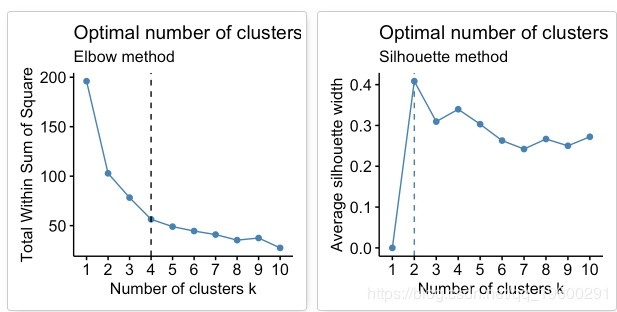​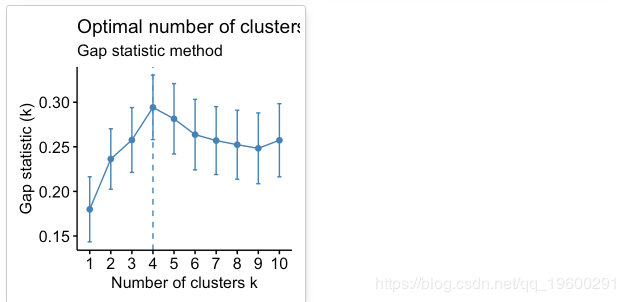​根据这些观察，有可能将k = 4定义为数据中的最佳簇数。30个索引，用于选择最佳数目的群集数据：矩阵diss：要使用的相异矩阵。默认情况下，diss = NULL，但是如果将其替换为差异矩阵，则距离应为“ NULL”distance：用于计算差异矩阵的距离度量。可能的值包括“ euclidean”，“ manhattan”或“ NULL”。min.nc，max.nc：分别为最小和最大簇数要为kmeans 计算NbClust（），请使用method =“ kmeans”。要计算用于层次聚类的NbClust（），方法应为c（“ ward.D”，“ ward.D2”，“ single”，“ complete”，“ average”）之一。下面的R代码为k均值计算 ：## Among all indices:
## ===================
## * 2 proposed  0 as the best number of clusters
## * 10 proposed  2 as the best number of clusters
## * 2 proposed  3 as the best number of clusters
## * 8 proposed  4 as the best number of clusters
## * 1 proposed  5 as the best number of clusters
## * 1 proposed  8 as the best number of clusters
## * 2 proposed  10 as the best number of clusters
##
## Conclusion
## =========================
## * According to the majority rule, the best number of clusters is  2 .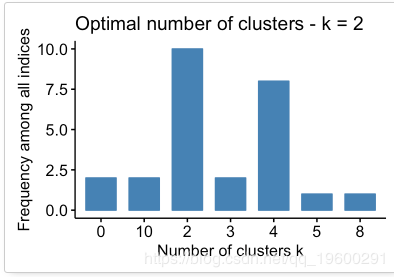​根据多数规则，最佳群集数为2。
展开全文• 论文研究-模糊C-均值中的最优聚类与最佳聚类数.pdf, 根据模糊 C-均值(FCM)算法中的类中距与类间距构造一个新且简单的分类准则函数vZS ,利用迭代自组织分析技术(ISODATA)...
• 文章目录主要内容确定最佳聚类数 本课程来自深度之眼，部分截图来自课程视频以及李航老师的《统计学习方法》第二版。 公式输入请参考：在线Latex公式 主要内容 确定最佳聚类数初步方法：理解给定阈值法，数据点散布...
文章目录主要内容确定最佳聚类数1、给定阈值2、数据点散布图3、根据统计量分析聚类数量预备知识$R^2$统计量半偏$R^2$统计量伪F统计量伪$t^2$统计量谱系图
本课程来自深度之眼，部分截图来自课程视频以及李航老师的《统计学习方法》第二版。
公式输入请参考：在线Latex公式
主要内容
确定最佳聚类数初步方法：理解给定阈值法，数据点散布图法
总离差平方和的分解：SST=SSA+SSE
聚类相关统计量：$R^2$统计量定义与表达式理解
统计量法：半偏$R^2$统计量：半偏$R^2$统计量定义与表达式理解
统计量法：伪F统计量：伪F统计量定义与表达式理解
统计量法：伪$t^2$统计量：伪$t^2$统计量定义与表达式理解
谱系图法：根据谱系图进行分类的准则
伪F统计量案例分析：观察伪F统计量值与分类数的散点图，分析聚类树形图
确定最佳聚类数
选定某种聚类方法，按系统聚类的步骤并类后，得到谱系聚类图，反映样本（或变量）间的亲属关系。
1、给定阈值
给出合适的阀值$T$，当类间距离$\le T$时，形成的各个类中所包含的样品间关系密切，应属于同一类；反之不并类。
2、数据点散布图
a.若考察2个指标，可通过数据点的散布图直观确定类的个数。
b.若考察3个指标，可通过绘制三维散布图，旋转三维坐标轴，由数据点的分布确定应分为几个类。
c.若考察3个以上指标，可由这些指标综合出2个或3个综合变量，绘制数据点在综合变量上的散布图。
3、根据统计量分析聚类数量
预备知识
总离差平方和（Sum of Squares Total）
残差平方和（Sum of Squared Errors）
回归平方和（Sum of Squares Regression ）
组间离差平方和 (Sum of Squares for factor A)
这里关于总离差平方和的分解简单推导一下：
$\sum_{i=1}^n(y_i-\bar y)^2=\sum_{k=1}^K\sum_{i=1}^{n_k}(y_i^{(k)}-\bar y^{(k)})^2+\sum_{k=1}^K\sum_{i=1}^{n_k}(\bar y^{(k)}-\bar y)^2$
右边第一项第k类里面的第i项$y_i^{(k)}$减去该类平均值$\bar y^{(k)}$，然后累加该类的$n_k$项，累加K个类；第二项是第k类平均值$\bar y^{(k)}$减去总平均值$\bar y$，然后累加该类的$n_k$项，累加K个类。
本来右边是：
$\sum_{k=1}^K\sum_{i=1}^{n_k}(y_i^{(k)}-\bar y^{(k)}+\bar y^{(k)}-\bar y)^2$
两两看成一项展开：
$\sum_{k=1}^K\sum_{i=1}^{n_k}(y_i^{(k)}-\bar y^{(k)})^2+\sum_{k=1}^K\sum_{i=1}^{n_k}(\bar y^{(k)}-\bar y)^2\\ +2\sum_{k=1}^K\sum_{i=1}^{n_k}(y_i^{(k)}-\bar y^{(k)})(\bar y^{(k)}-\bar y)$
在计算内层累加的时候$(\bar y^{(k)}-\bar y)$不用累加（没有i），只用算前面一项：
$\sum_{i=1}^{n_k}(y_i^{(k)}-\bar y^{(k)})=\sum_{i=1}^{n_k}y_i^{(k)}-\sum_{i=1}^{n_k}\bar y^{(k)}\\ =n_k\bar y^{(k)}-n_k\bar y^{(k)}=0$
所以交叉项为0.
有了这个结论，我们来看如果有一个矩阵，其每个列都是一个类：
$\begin{bmatrix} x_{11} & x_{12} &\cdots &x_{1p} \\ x_{21} & x_{22} & \cdots & x_{2p}\\ \vdots & \vdots & \ddots & \vdots\\ x_{n1} &x_{n2} &\cdots &x_{np} \end{bmatrix}\\\begin{matrix} \bar x_1 & \bar x_2 & \cdots& \bar x_p \end{matrix}$
那么这个矩阵的总离差平方和的分解为（各个类减去该类均值）：
$(x_{11}-\bar x_1)^2+\cdots+(x_{n1}-\bar x_1)^2+\cdots+(x_{1p}-\bar x_p)^2+\cdots+(x_{np}-\bar x_p)^2$
把总的影响平方和记为SST，它分为两个部分，一部分是由控制变量引起的离差，记为SSA(组间离差平方和)，另一部分是由随机变量引起的SSE（组内离差平方和）。
即SST=SSA+SSE。
F记为
$F=\cfrac{SSA/(k-1)}{SSE/(n-k)}$
那么它是服从分布$F(k-1,n-k)$
当数据集的F大于上面的$F_\alpha$表明组间离差平方和较大（k个类的差异较大）。反之类间的差异不明显。
$R^2$统计量
$R^2=\cfrac{SSR}{SST}=\cfrac{\sum_{i=1}^n(\hat y_i-\bar y)^2}{\sum_{i=1}^n(y_i-\bar y)^2}$
$\hat y_i,y_i$分别是估计值和真实值，如果预测准确，二者非常接近，则$R^2$趋近于1。
假定数据集已将样本分为k类，$G_1,G_2,\cdots,G_k$，第i类含样品数$n_i$，且有：$n_1+n_2+\cdots+n_k=n$，记$G_t$重心为$\bar X^{(t)}$，$G_t$中第i个样本为：$\bar X^{(t)}_{(i)},(i=1,2,\cdots,n_t)$，所有样本的重心为$\bar X$，则$G_t$类中$n_t$个样本的离差平方和为：
$W_t=\sum_{i=1}^{n_t}(\bar X^{(t)}_{(i)}-\bar X^{(t)})^T(\bar X^{(t)}_{(i)}-\bar X^{(t)})$
所有样本SST：
$SST=\sum_{t=1}^k\sum_{i=1}^{n_t}(\bar X^{(t)}_{(i)}-\bar X^{(t)})^T(\bar X^{(t)}_{(i)}-\bar X^{(t)})$
SST可以分解(上面的形式是：SST=SSA+SSE)：
$SST=\sum_{t=1}^kW_t+B_k=P_k+B_k$
令$R^2_k=\cfrac{B_k}{SST}=1-\cfrac{P_k}{SST}$
$R^2$越大，则类间差异越明显。
半偏$R^2$统计量
$半偏R^2=\cfrac{B_{KL}^2}{SST}=R^2_{k+1}-R^2_{k}$
如果某一个步骤的半偏$R^2$越大，说明这一步取消一个分类后（从k+1分类变成k类）的类间差异变小了，因此上一个步骤分为k+1个分类聚类效果好。
伪F统计量
$伪F_k=\cfrac{(SST-P_k)/(k-1)}{P_k/(n-k)}=\cfrac{B_k}{P_k}\cfrac{n-k}{k-1}$
因为我们不知道原总体理论分布是否为正态分布，因此$F_k$统计量并不精确服从F分布，若某步骤伪$F_k$统计量较⼤，则表明这n个样品可显著地分为k个类
伪$t^2$统计量
$伪t^2=\cfrac{B^2_{KL}}{(W_K+W_L)/(n_K+n_L-2)}$
若某步骤伪$t^2$统计量值较大，则表明$G_K$和$G_L$合并为$G_{KL}$后类内离差平方和增量$B^2_{KL}$比$G_K$和$G_L$合并前的类内离差平方和大，那么就说明上一步没有合并前的$G_K$和$G_L$两个类的类间差异较大，不应该合并为$G_{KL}$。
谱系图
Bermirmen(1972）提出根据谱系图进⾏分类的准则：
a.各类重心间距离较远
b.确定的类中各类包含元素不宜多
c.分类数符合研究目的
d.若运⽤集中不同的聚类方法处理，则应在各自的聚类图中发现相同的类


展开全文• @在R语言中用 elbow 方法确定最佳聚类数录 如何用在R语言中用 elbow 方法确定最佳聚类数 在无监督学习中，我们有时候使用 k-means 方法进行聚类，对数据进行分析。k-means 很好用，但是确定最佳类别数是一个需要技术...
• 根据方差分析理论，提出应用混合F统计量来确定最佳分类数，并应用模糊划分熵来验证最佳分类数的正确性，综合运用上述两个指标可以准确确定最佳聚类数。通过实际算例说明这两个指标的有效性。
• 挖掘过程中用到了K-means聚类方法，但是由于根据行业经验确定的聚类数过多并且并不一定是我们获取到数据的真实聚类数，所以，我们希望能从数据自身出发去确定真实的聚类数，也就是对数据而言的最佳聚类数。...
• 新的K-均值算法最佳聚类数确定方法,对均值聚类算法有极大地优化
• 聚类中也优化的ｋ－ｍｅａｎｓ最佳聚类数确定方法1 获得初始中心点候选集对ｋ－ｍｅａｎｓ算法初始中心点的选择，要求选取的中也点能很好的反映数据集中对象之间的关系和数据的分布特征，这对聚类结果的准确性和稳定...
• 无监督ML预测 从给定的“虹膜”数据集中，预测最佳聚类数并直观地表示出来。
• KMeans算法和Elbow准则 “ k-Means聚类背后的想法是获取一堆数据并确定数据中是否存在任何自然聚类（相关对象的组）。 k-Means算法是所谓的无监督学习算法。... 使用“肘标准”为K-means算法确定最佳聚类数
• 使用DBSCAN标识为员工分组照片由Ishan @seefromthesky 在 Unsplash拍摄基于密度的噪声应用空间聚类(DBSCAN)是一种无监督的ML聚类算法。无监督的意思是它不使用预先标记的目标来聚类数据点。聚类是指试图将相似的数据...
• 为验证在聚类分析的聚类结果,重要的是要使用一些客观评价聚类质量的指标。本matlab工具箱提供4个外部效度指标和8个内部效度指标: Rand index, Adjusted Rand index, Silhouette, Calinski-Harabasz, Davies-Bouldin,...
• 参考：https://stackoom.com/question/12W1D/R中的聚类分析-确定最佳聚类数 #2楼 If your question is how can I determine how many clusters are appropriate for a kmeans analysis of my data? 如果您的问题是...r k-means
• 转载请注明出处，该文章的官方来源：快速迭代聚类 | Teaching ML1 谱聚类算法的原理 在分析快速迭代聚类之前，我们先来了解一下谱聚类算法。谱聚类算法是建立在谱图理论的基础上的算法，与传统的聚类算法相比，它能...
• k均值聚类用于大数据 介绍 (Introduction) K-means is one of the most widely used unsupervised clustering methods. K均值是最广泛使用的无监督聚类方法之一。 The K-means algorithm clusters the data at hand ...python java 机器学习 linux 大数据
• 三维数据的k均值聚类介绍(Introduction) K-means is one of the most widely used unsupervised clustering methods.K均值是最广泛使用的无监督聚类方法之一。The K-means algorithm clusters the data at hand by ...python java 大数据 机器学习 人工智能
• 关键点：National Health and Nutrition Exam Survey (综合饮食，医学和检查机器学习数据集，2013年至2014年)中有一些聚类，这些聚类只能通过降维来显示。PCA与k-means结合使用是一种可视化高维数据的强大方法。本文......